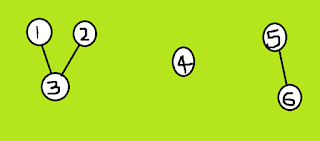Given a connected undirected graph. Perform a Depth First Traversal of the graph.

Example 1:

```there are 3 connected components in the above undirected graph
1-2-3
4
5-6
```

method:

1) Initialize all vertices as not visited.

in  dfs function for every vertex 'v',if 'v' is not visited before, call dfsui(v)

2)in dfsui(v),Mark 'v' as visited.

for every adjacent 'u' of 'v',

If 'u' is not visited, then recursively call dfsui(u)

if adjacent nodes exit than it goes recursively ,

once the adjacent nodes are not there it comes out of dfsui()

and goes back to dfs() which describes that it was one of the connected component.

now in dfs we increment the counter and again every vertex 'v' is checked again.

c++ implementation:

```void dfsui(int source, vector<int> g[], bool vis[])
{
vis[source] = true;

for(auto i:g[source])
{
if(!vis[i])
dfsui(i, g, vis);
}

}

int dfs(vector<int> g[], int N)
{
bool vis[N]; int c=0;
for(int i=0;i<N;i++)
vis[i]=0;

int k;
for(k=0;k<N;k++)
{
if(vis[k]==0)
{
dfsui(k,g,vis);
c++;
}
}return c;
}```

• Time complexity: O(V + E), where V is the number of vertices and E is the number of edges in the graph.
• Space Complexity :O(V).
Since an extra visited array is needed of size V.
darkmode